Test: Multiplexers

# Test: Multiplexers

Test Description

## 14 Questions MCQ Test GATE Computer Science Engineering(CSE) 2023 Mock Test Series | Test: Multiplexers

Test: Multiplexers for Computer Science Engineering (CSE) 2023 is part of GATE Computer Science Engineering(CSE) 2023 Mock Test Series preparation. The Test: Multiplexers questions and answers have been prepared according to the Computer Science Engineering (CSE) exam syllabus.The Test: Multiplexers MCQs are made for Computer Science Engineering (CSE) 2023 Exam. Find important definitions, questions, notes, meanings, examples, exercises, MCQs and online tests for Test: Multiplexers below.
Solutions of Test: Multiplexers questions in English are available as part of our GATE Computer Science Engineering(CSE) 2023 Mock Test Series for Computer Science Engineering (CSE) & Test: Multiplexers solutions in Hindi for GATE Computer Science Engineering(CSE) 2023 Mock Test Series course. Download more important topics, notes, lectures and mock test series for Computer Science Engineering (CSE) Exam by signing up for free. Attempt Test: Multiplexers | 14 questions in 42 minutes | Mock test for Computer Science Engineering (CSE) preparation | Free important questions MCQ to study GATE Computer Science Engineering(CSE) 2023 Mock Test Series for Computer Science Engineering (CSE) Exam | Download free PDF with solutions
 1 Crore+ students have signed up on EduRev. Have you?
Test: Multiplexers - Question 1

### Consider the following Boolean function of four variables: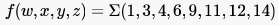The function is

Detailed Solution for Test: Multiplexers - Question 1

The K-map would be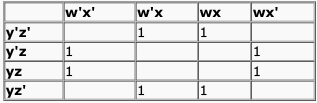So, the minimized expression would
be x'z + xz'.
So, option B.

Test: Multiplexers - Question 2

### Consider the following Boolean function of four variables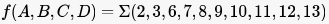The function is

Detailed Solution for Test: Multiplexers - Question 2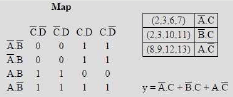Test: Multiplexers - Question 3

### The simplified SOP (Sum of Product) from the Boolean expression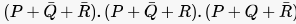1s

Detailed Solution for Test: Multiplexers - Question 3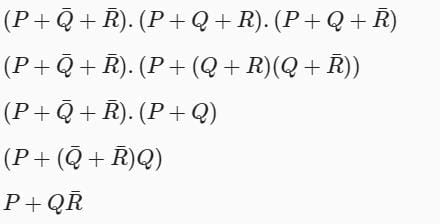Test: Multiplexers - Question 4

Consider the 4-to-1 multiplexer with two select lines S1 and S0 given below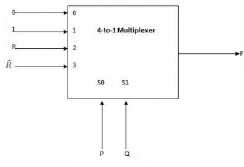The minimal sum-of-products form of the Boolean expression for the output F of the multiplexer is

Detailed Solution for Test: Multiplexers - Question 4

S0 and S1 are used to select the input given to be given as output.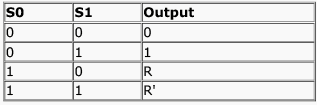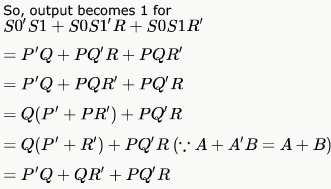Test: Multiplexers - Question 5

Consider the following Boolean expression for F: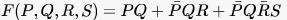The minimal sum of products form of F is

Detailed Solution for Test: Multiplexers - Question 5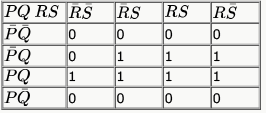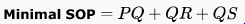Hence, option A is correct.

Test: Multiplexers - Question 6

Consider the following minterm expression F for :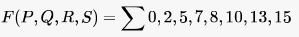The minterms 2, 7, 8 and 13 are 'do not care' terms. The minimal sum-of-products form for F is

Detailed Solution for Test: Multiplexers - Question 6

While putting the terms to K-map the 3rd and 4th columns are swapped so do 3rd and 4th rows. So, term 2 is going to (0,3) column instead of (0,2), 8 is going to (3,0) instead of (2,0) etc..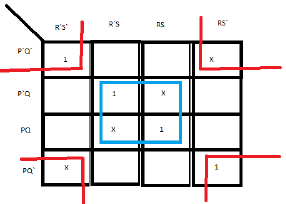Solving this k-map gives B) as the answer.

Test: Multiplexers - Question 7

Consider the two cascade 2 to 1 multiplexers as shown in the figure .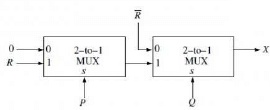The minimal sum of products form of the output X is

Detailed Solution for Test: Multiplexers - Question 7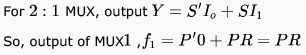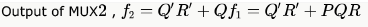Test: Multiplexers - Question 8

The output F of the below multiplexer circuit can be represented by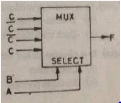Detailed Solution for Test: Multiplexers - Question 8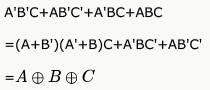Test: Multiplexers - Question 9

A multiplexer with a 4 - bit data select input is a

Detailed Solution for Test: Multiplexers - Question 9

for n bit data select input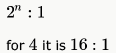Test: Multiplexers - Question 10

It is possible for an enable or strobe input to undergo an expansion of two or more MUX ICs to the digital multiplexer with the proficiency of large number of

Detailed Solution for Test: Multiplexers - Question 10

It is possible for an enable or strobe input to undergo an expansion of two or more MUX ICs to the digital multiplexer with the proficiency of large number of inputs.

Test: Multiplexers - Question 11

Consider a multiplexer with X and Y as data inputs and Z the as control input. Z = 0 selects input X, and Z = 1 selects input Y. What are the connections required to realize the 2-variable Boolean function F = T + R, without using any additional hardware?

Detailed Solution for Test: Multiplexers - Question 11

Z'X + ZY..
Put Z = T, X = R, Y = 1 in Z'X + ZY..
= T'R + 1*T
= (T+T') (T+R)
= T + R

Test: Multiplexers - Question 12

The circuit shown below implements a 2-input NOR gate using two 2  4 MUX (control signal 1 selects the upper input).
What are the values of signals x,y and z?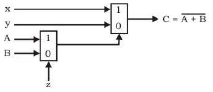Detailed Solution for Test: Multiplexers - Question 12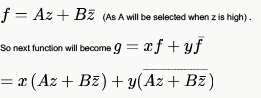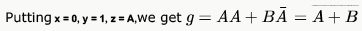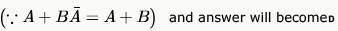Test: Multiplexers - Question 13

Suppose only one multiplexer and one inverter are allowed to be used to implement any Boolean function of  variables.
What is the minimum size of the multiplexer needed?

Detailed Solution for Test: Multiplexers - Question 13

2n - 1
We will map  variables  to select lines and  variable to input line

Test: Multiplexers - Question 14

The following circuit implements a two-input AND gate using two 2-1 multiplexers.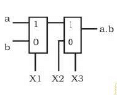What are the values of X1,X2,X3?

Detailed Solution for Test: Multiplexers - Question 14

F = (bX1' + aX1)X3 + X2X3'
Put X1 = b, X2 = 0, X3 = a to get F = ab.

## GATE Computer Science Engineering(CSE) 2023 Mock Test Series

150 docs|215 tests
 Use Code STAYHOME200 and get INR 200 additional OFF Use Coupon Code